## Using Elementary Row Operations to Determine A−1

A linear system is said to be square if the number of equations matches the number of unknowns. If the system A x = b is square, then the coefficient matrix, A, is square. If A has an inverse, then the solution to the system A x = b can be found by multiplying both sides by A −1: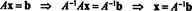This calculation establishes the following result:

Theorem D. If A is an invertible n by n matrix, then the system A x = b has a unique solution for every n‐vector b, and this solution equals A −1 b.

Since the determination of A −1 typically requires more calculation than performing Gaussian elimination and backsubstitution, this is not necessarily an improved method of solving A x = b (And, of course, if A is not square, then it has no inverse, so this method is not even an option for nonsquare systems.) However, if the coefficient matrix A is square, and if A −1 is known or the solution of A x = b is required for several different b's, then this method is indeed useful, from both a theoretical and a practical point of view. The purpose of this section is to show how the elemenetary row operations that characterize Gauss‐Jordan elimination can be applied to compute the inverse of a square matrix.

First, a definition: If an elementary row operation (the interchange of two rows, the multiplication of a row by a nonzero constant, or the addition of a multiple of one row to another) is applied to the identity matrix, I, the result is called an elementary matrix. To illustrate, consider the 3 by 3 identity matrix. If the first and third rows are interchanged,or if the second row of I is multiplied by −2,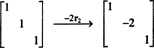or if −2 times the first row is added to the second row,all of these resulting matrices are examples of elementary matrices. The first fact that will be needed to compute A −1 reads as follows: If E is the elementary matrix that results when a particular elementary row operation is performed on I, then the product EA is equal to the matrix that would result if that same elementary row operation were applied to A. In other words, an elementary row operation on a matrix A can be performed by multiplying A on the left by the corresponding elementary matrix. For example, consider the matrix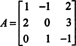Adding −2 times the first row to the second row yields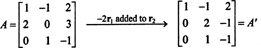If this same elementary row operation is applied to I,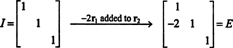then the result above guarantees that EA should equal A′. You may verify that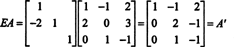is indeed true.

If A is an invertible matrix, then some sequence of elementary row operations will transform A into the identity matrix, I. Since each of these operations is equivalent to left multiplication by an elementary matrix, the first step in the reduction of A to I would be given by the product E 1 A, the second step would be given by E 2 E 1 A, and so on. Thus, there exist elementary matrices E 1, E 2,…, E k such that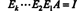But this equation makes it clear that E kE 2 E 1 = A −1: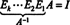Since E kE 2 E 1 = E kE 2 E 1 I, where the right‐hand side explicitly denotes the elementary row operations applied to the identity matrix I, the same elementary row operations that transform A into I will transform I into A −1. For n by n matrices A with n > 3, this describes the most efficient method for determining A −1.

Example 1: Determine the inverse of the matrix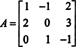Since the elementary row operations that will be applied to A will be applied to I as well, it is convenient here to augment the matrix A with the identity matrix I:Then, as A is transformed into I, I will be transformed into A −1:Now for a sequence of elementary row operations that will effect this transformation: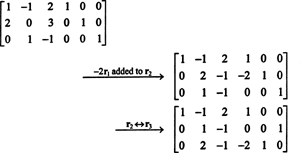Since the transformation [ A | I] → [ I | A −1] reads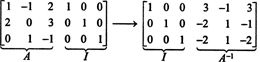the inverse of the given matrix A is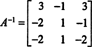Example 2: What condition must the entries of a general 2 by 2 matrixsatisfy in order for A to be invertible? What is the inverse of A in this case?

The goal is to effect the transformation [ A | I] → [ I | A −1]. First, augment A with the 2 by 2 identity matrix: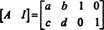Now, if a = 0, switch the rows. If c is also 0, then the proces of reducing A to I cannot even begin. So, one necessary condition for A to be invertible is that the entries a and c are not both 0. Assume that a ≠ 0. Then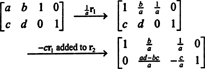Next, assuming that adbc ≠ 0,Therefore, if adbc ≠ 0, then the matrix A is invertible, and its inverse is given by(The requirement that a and c are not both 0 is automatically included in the condition adbc ≠ 0.) In words, the inverse is obtained from the given matrix by interchanging the diagonal entries, changing the signs of the off‐diagonal entries, and then dividing by the quantity adbc. This formula for the inverse of a 2 x 2 matrix should be memorized.

To illustrate, consider the matrixSince adbc = (−2)(5) − (−3)(4) = 2 ≠ 0, the matrix is invertible, and its inverse is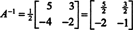You may verify thatand that A −1 A = I also.

Example 3: Let A be the matrix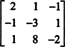Is A invertible?

No. Row reduction of A produces the matrix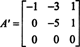The row of zeros signifies that A cannot be transformed to the identity matrix by a sequence of elementary row operations; A is noninvertible. Another argument for the noninvertibility of A follows from the result Theorem D. If A were invertible, then Theorem D would guarantee the existence of a solution to A x = b for every column vector b = ( b 1, b 2, b 3) T. But A x = b is consistent only for those vectors b for which b 1 + 3 b 2 + b 3 = 0. Clearly, then, there exist (infinitely many) vectors b for which A x = b is inconsistent; thus, A cannot be invertible.

Example 4: What can you say about the solutions of the homogeneous system A x = 0 if the matrix A is invertible?

Theorem D guarantees that for an invertible matrix A, the system A x = b is consistent for every possible choice of the column vector b and that the unique solution is given by A −1 b. In the case of a homogeneous system, the vector b is 0, so the system has only the trivial solution: x = A −1 0 = 0.

Example 5: Solve the matrix equation AX = B, whereSolution 1. Since A is 3 x 3 and B is 3 x 2, if a matrix X exists such that AX = B, then X must be 3 x 2. If A is invertible, one way to find X is to determine A −1 and then to compute X = A −1 B. The algorithm [ A | I] → [ I | A −1] to find A −1 yields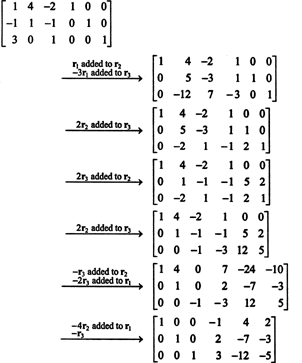Therefore,so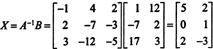Solution 2. Let b 1 and b 2 denote, respectively, column 1 and column 2 of the matrix B. If the solution to A x = b 1 is x 1 and the solution to A x = b 2 is x 2, then the solution to AX = B = [ b 1 b 2] is X = [ x 1 x 2]. That is, the elimination procedure can be performed on the two systems ( A x = b 1 and A x = b 2)

simultaneously:Gauss‐Jordan elimination completes the evaluation of the components of x 1 and x 2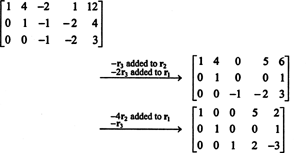It follows immediately from this final augmented matrix that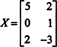as before.

It is easy to verify that the matrix X does indeed satisfy the equation AX = B:Note that the transformation in Solution 1 was [ A | I] → [ I | A −1], from which A −1 B was computed to give X. However, the transformation in Solution 2, [ A | B] → [ I | X], gave X directly.